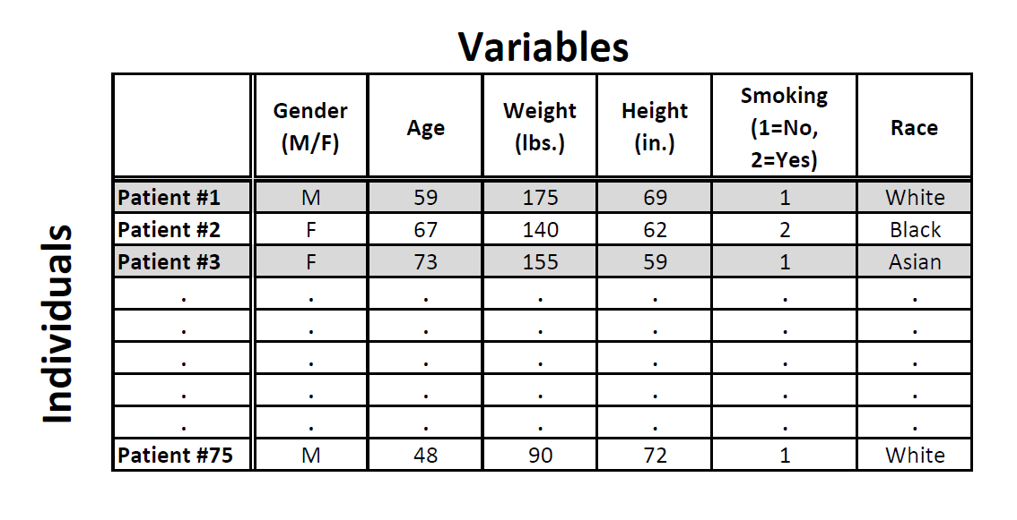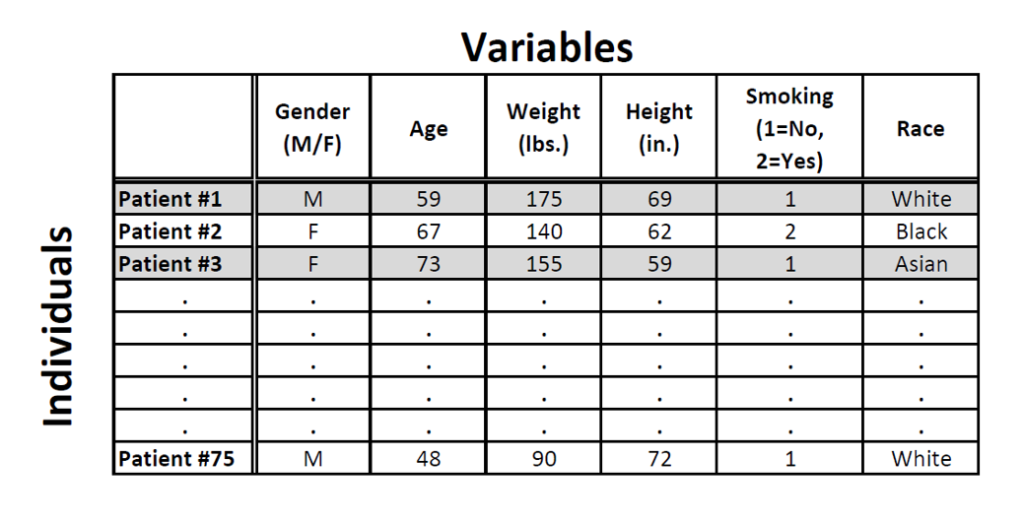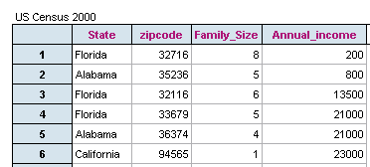• by Team Handson
• September 20, 2022

## Exploratory Data Analysis

What do mean by Exploratory Data Analysis?

• Exploratory Data Analysis (EDA) is used to summarize the data we have gathered in a meaningful way. This helps us to get the necessary insight about the data.
• The tools, tricks and rules to summarize the collected data are all part of EDA.

Why EDA is Necessary?

• EDA is an important part of statistical analysis (also called descriptive statistics).
• It helps us in understanding and visualizing the collected data.
• EDA is useful for summarizing various facts. For example, to summarize the results of students in a school / college, it is used.## Data and Variables:

What is Data?

• Data are pieces of information about individuals that organized into variables.
• By an individual (also called record), we mean a particular person or object.
• By a variable, we mean a particular characteristic of the individual.

The following dataset displays medical records from a specific survey:Usually, variables are arranged across columns while the individuals (records) are arranged across rows

What are Variables?

Variables or Data Items represent any number, quality or characteristics. Variables can be categorized into two types: categorical or quantitative.

• Categorical Variable (Qualitative Variable): It takes category or label values and places an individual into one of several groups. Each observation can be placed in only one category. The categories are mutually exclusive.
• Numerical Variable (Quantitative Variable): It takes numerical values and represent some kind of measurement.In our example:

• Gender and Smoking are categorical variables.
• AgeWeight and Height are quantitative variables.

We took a random data from the 2000 U.S. Census. Here is part of the dataset:Q.1. Who are the individuals described by this data?

Ans: States (People living in the United States in the year 2000) and People with families in the year 2000.

Q.2. What type of variable is Zip code?

Ans: Categorical or Qualitative

Q.3. What type of variable is Annual Income?

Ans: Numerical or Quantitative

• Zip code is a categorical variable because it categorizes individuals by geographic location
• Annual Income is a Quantitative variable because it assumes continuous values over a range and has arithmetic significance.